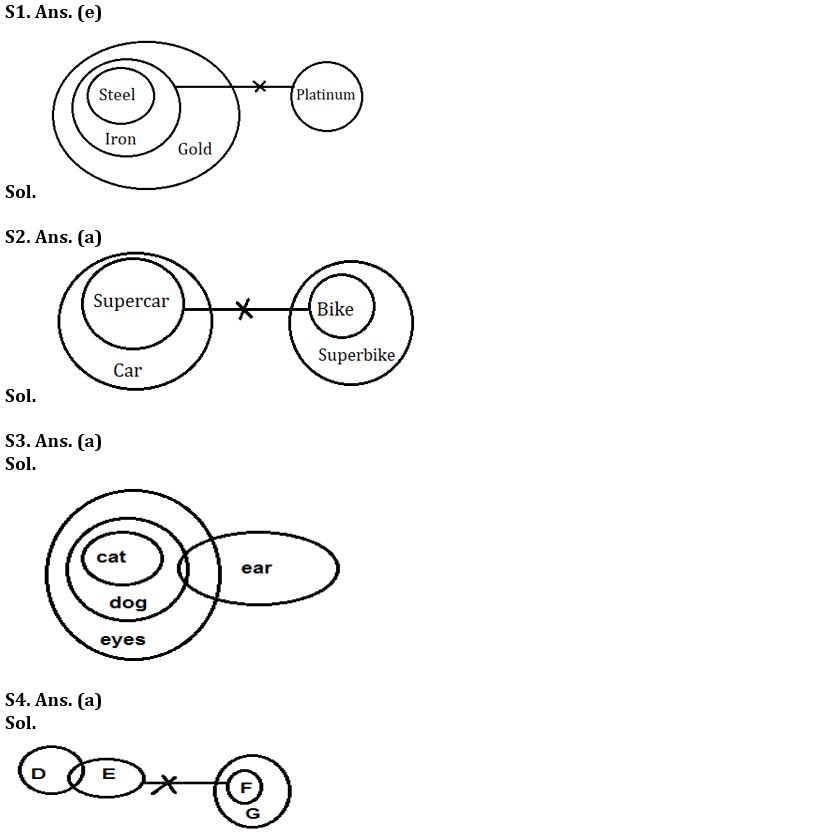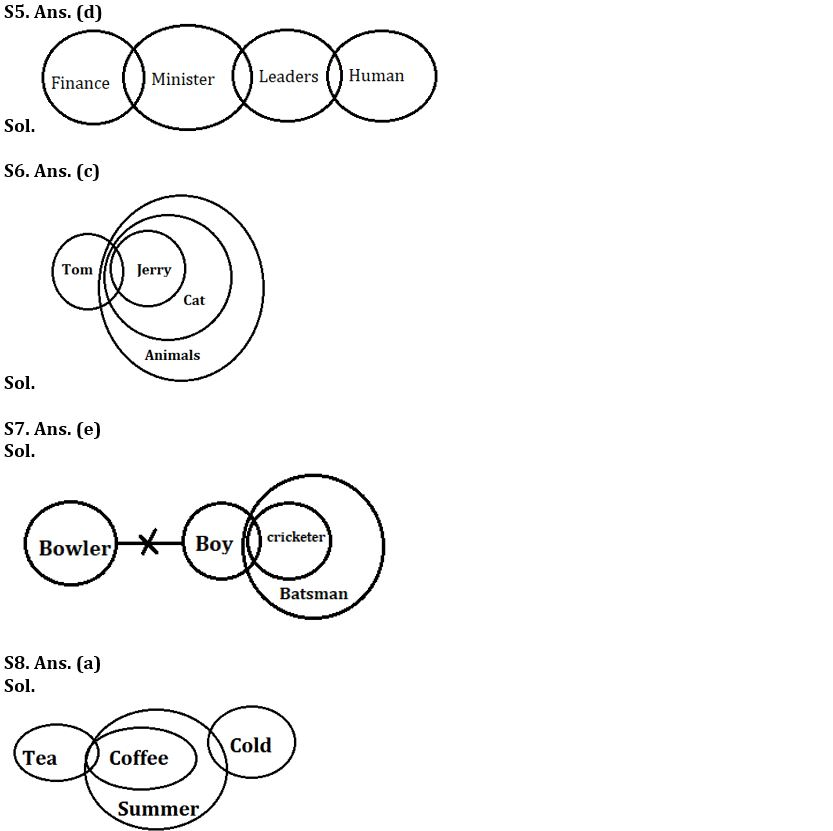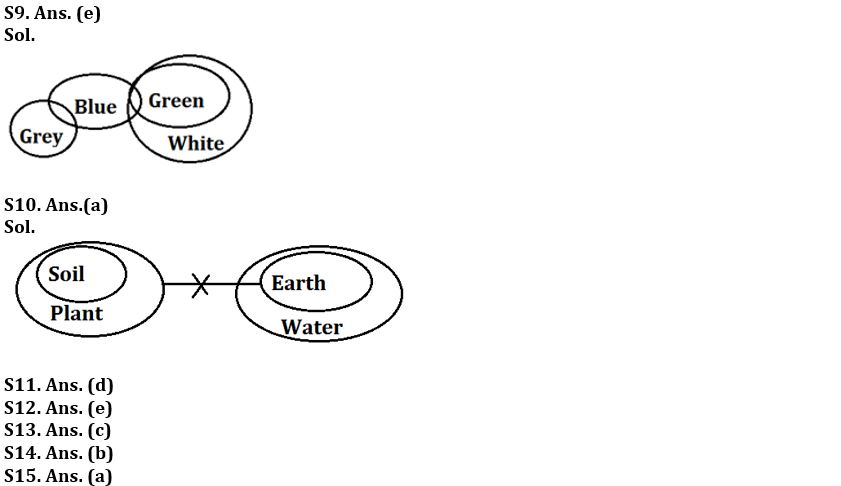Latest Banking jobs   »

# Reasoning Ability Quiz For SBI PO Prelims 2022- 30th November

Directions (1-5): In each of the questions below some statements are given followed by some Conclusions. You have to take the given statements to be true even, if they seem to be at variance from commonly known facts. Read all the conclusions and then decide which of the given conclusions logically follows from the given statements disregarding commonly known facts.
(a) If only conclusion I follows.
(b) If only conclusion II follows.
(c) If either conclusion I or II follows.
(d) If neither conclusion I nor II follows.
(e) If both conclusions I and II follow.

Q1. Statements: All Steel are Iron
All Iron are Gold
No Iron is Platinum
Conclusion: I: Some Gold are not Platinum
II: Some Steel are not Platinum

Q2. Statements: All Supercar is Car
No Supercar is Bike
All bike are Superbike
Conclusion: I:All Car can never be Bike
II: Some Superbike is Supercar.

Q3. Statements: All cat are dog.
All dog are eyes.
Some dog are ear
Conclusions: I. All ear being eyes is a possibility
II. No cat is ear

Q4. Statements: Some D is E.
No E is a F.
All F are G
Conclusions: I. Some G are definitely not E
II. Some F are not D

Q5. Statements: Some Finance are Minister
Conclusions: I: All minister are Leader
II: Some Leaders are not Minister

Directions (6-10): In each of the questions below some statements are given followed by some Conclusions. You have to take the given statements to be true even, if they seem to be at variance from commonly known facts. Read all the conclusions and then decide which of the given conclusions logically follows from the given statements disregarding commonly known facts.
(a) If only conclusion I follows.
(b) If only conclusion II follows.
(c) If either conclusion I or II follows.
(d) If neither conclusion I nor II follows.
(e) If both conclusions I and II follow.

Q6. Statements: Some Tom are Jerry
All Jerry are Cat
All cat are Animal
Conclusions: I: Some Tom are not Animals
II: All Tom are animals

Q7. Statements: Some Boy are Cricketer
All Cricketer are Batsman
No Boy is Bowler
Conclusions: I: Some Cricketer is not bowler
II: All Batsman can never be bowler

Q8. Statements: All coffee are summer
Some tea is coffee
Some summer are cold
Conclusions: I. All tea being cold is a possibility
II. Some coffee are definitely not cold.

Q9. Statements: Some blue are green
Some grey is blue
All green are white
Conclusions: I. Some grey is white is a possibility
II. All green are grey is a possibility

Q10. Statements: All soil are plant
No earth is plant
All earth are water
Conclusions: I. Some plant is water is a possibility.
II. No water is soil

Directions (11-15): In this question, relationship between different elements is shown in the statements. The statements are followed by conclusions. Study the conclusions based on the given statement and select the appropriate answer.

Q11. Statements: L = P ≤ W < V ≤ K ≥ Q; B < L; K = M
Conclusions: I. B < V
II. M > P
(a) Only conclusion I is true
(b) Only conclusion II is true
(c) Either conclusion I or II is true
(d) Both conclusion I and II are true
(e) Neither conclusion I nor II is true

Q12. Statements: L = P ≤ W < V ≤ K ≥ Q; B < L; K = M
Conclusions: I. L ≥ Q
II. W = M
(a) Only conclusion I is true
(b) Only conclusion II is true
(c) Either conclusion I or II is true
(d) Both conclusion I and II are true
(e) Neither conclusion I nor II is true

Q13. Statements: R ≤ U = B < S; B ≤ X
Conclusions: I. X > R
II. X = R
(a) Only conclusion I is true
(b) Only conclusion II is true
(c) Either conclusion I or II is true
(d) Both conclusion I and II are true
(e) Neither conclusion I nor II is true

Q14. Statements: C > U ≤ S < T = O > D ≥ Y; Z = O ≤ P
Conclusions: I. U > D
II. S < P
(a) Only conclusion I is true
(b) Only conclusion II is true
(c) Either conclusion I or II is true
(d) Both conclusion I and II are true
(e) Neither conclusion I nor II is true

Q15. Statements: C > U ≤ S < T = O > D ≥ Y; Z = O ≤ P
Conclusions: I. Z > Y
II. C < O
(a) Only conclusion I is true
(b) Only conclusion II is true
(c) Either conclusion I or II is true
(d) Both conclusion I and II are true
(e) Neither conclusion I nor II is true

Solutions#### Congratulations!